# Understanding crosstalk effect between two parallel cables

#### clmoi90

Joined Mar 29, 2018
9
Dear,

I am trying to understand the impact of crosstalk between two "parallel" cables. I have searched information online and my first understanding of crosstalk is as follows:
With this understanding, I could make some assumptions:
• Higher current variation will imply higher magnetic field variation and so higher crosstalk coupling
• A square signal will have higher crosstalk coupling than a sinus (sharp edges)
I wanted to analyse the impact of the distance between both cables in the crosstalk coupling so I tried to go into equation to compute crosstalk effect.
I found this equation for the crosstalk voltage: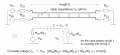And this equation for the calculation of the parallel line capacitance C: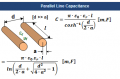With Epsilon_r = 1.0006 (permitivity of air (not really true since I should also consider the permitivity of the skin of the cable)) and Epsilon_0 = 8.85 x 10-12 F/m (permittivity of free space).

I have input these equations on an excel sheet (attached if anyone need it) and tried to play with different parameters to see their impact but crosstalk is not clear anymore for me (I naively thought it was)... My issues are:
• In these equations I don't see any link with the fact that the crosstalk coupling happens during a variation, shouldn't we have an integral somewhere in the equation?
• Since no integral in the equation, I don't see how the shape of the disturbing signal impact the crosstalk coupling
• Also it doesn't show how a higher current would have a higher crosstalk coupling
Anyone could enlighten me if I am not using the right equations or if I have misunderstood how crosstalk is created?

Best regards,
Ludovic

#### Attachments

• 66.4 KB Views: 7

#### ScottWang

Joined Aug 23, 2012
6,829
Could you upload the file as *.xls or *.pdf, maybe our helpers don't have the new software to open it and I'm the one can't open the file, if I want to open it then I have to install a software can open it.

#### MrChips

Joined Oct 2, 2009
19,379
The shape of the signal is embedded in ω. Different signal shapes will have different frequency components.

The crosstalk voltage Vx is a function of Vs1. Higher current gives higher Vs1.

#### clmoi90

Joined Mar 29, 2018
9
Thank you for your replies but It's still not clear for me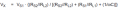About the shape of the signal:
What if we have one square signal of 500kHz (only 1 frequency) and one sinus signal of 500Khz (only 1 frequency). Then in both case ω = 2*pi*f is the same value and the crosstalk voltage Vx using above formula would be the same, right? But this is not inline with my understanding which is: higher slope variation in current will imply higher crosstalk coupling (in time domain). So I am lostLet's say we have two times the same circuit (circuit A and circuit B), only Rl1 would be different between both circuits with Rl1_A = 100kΩ, Rl1_B=10kΩ. Vs1 is same in both circuits so I1_B = 10*I1_A .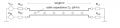If the source load is low (Rs1=100Ω), then Rs1_B//Rl1_B ≅ 99Ω and Rs1_A//Rl1_A ≅ 99.9Ω. The other parts of Vx equation would be same since the circuits A and B are same.
Thus the formula gives a crosstalk coupling very similar whereas there is a factor 10 between the current of both circuits.
So I still don't understand how the current level impacts the crosstalk coupling level...

I guess I don't get something, anyone could point out where I am wrong?

Best Regards,
Ludovic

#### noweare

Joined Jun 30, 2017
107
H=0.4*pi* Turns * amps/ (magnetic path length )

The h field is the driving force and gives rise to the lines of flux that links the victim cable.
For your example the H field is caused by 1 turn of wire ( the straight wire) multiplied by the changing current.
The H field is larger due to a larger current and would cause more interference.

#### Danko

Joined Nov 22, 2017
817
About the shape of the signal:
What if we have one square signal of 500kHz (only 1 frequency) and one sinus signal of 500Khz (only 1 frequency). Then in both case ω = 2*pi*f is the same value and the crosstalk voltage Vx using above formula would be the same, right? But this is not inline with my understanding which is: higher slope variation in current will imply higher crosstalk coupling (in time domain). So I am lostSquare signal 500kHz is mix of many different frequencies sinus signals. 500kHz is property of lowest frequency sinus signal from mix.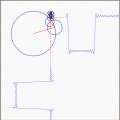#### clmoi90

Joined Mar 29, 2018
9
H=0.4*pi* Turns * amps/ (magnetic path length )

The h field is the driving force and gives rise to the lines of flux that links the victim cable.
For your example the H field is caused by 1 turn of wire ( the straight wire) multiplied by the changing current.
The H field is larger due to a larger current and would cause more interference.
I do agree that the magnetic field should somehow appear in the equation to calculate crosstalk coupling.
So is the formula not right? Or maybe limited to some applications?I am trying to calculate the impact of the distance between two cables on the crosstalk coupling but I don't know how to link the magnetic field to this calculation.

#### clmoi90

Joined Mar 29, 2018
9
Square signal 500kHz is mix of many different frequencies sinus signals. 500kHz is property of lowest frequency sinus signal from mix.
View attachment 150086
Do you mean, even if a micro-controller is outputing a PWM signal from a digital output, this PWM will actually be composed of different frequencies sinus signals? As it is shown on your picture.
So when calculating the crosstalk coupling, should I also consider these higher frequency harmonics? Higher is the signal frequency higher will be the crosstalk coupling, but in this PWM signal the high frequency harmonics would have very low amplitude.

#### Danko

Joined Nov 22, 2017
817
Do you mean, even if a micro-controller is outputing a PWM signal from a digital output, this PWM will actually be composed of different frequencies sinus signals? As it is shown on your picture.
Any square signal contains harmonics in the same proportions, independently from signal origin.
So when calculating the crosstalk coupling, should I also consider these higher frequency harmonics? Higher is the signal frequency higher will be the crosstalk coupling, but in this PWM signal the high frequency harmonics would have very low amplitude.
Of course you should, because crosstalk fast spike from square signal slope is sum of amplitudes of all harmonics.
Edit:
Check this link, maybe it will helps you in crosstalk calculation:
http://www.ivorcatt.com/6_3.htm

Last edited:

#### MrChips

Joined Oct 2, 2009
19,379
About the shape of the signal:
What if we have one square signal of 500kHz (only 1 frequency) and one sinus signal of 500Khz (only 1 frequency). Then in both case ω = 2*pi*f is the same value and the crosstalk voltage Vx using above formula would be the same, right? But this is not inline with my understanding which is: higher slope variation in current will imply higher crosstalk coupling (in time domain). So I am lostIf the square signal of 500kHz has only one frequency, what makes the shape different from a sine signal of 500kHz?
In other words, a signal with only one frequency must look like a sine signal.(Odd) harmonics of a 1000Hz square wave (Wikipedia)